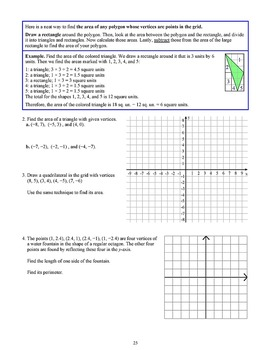digital

# Geometry, Grade 6 (Distance Learning)Subject
Resource Type
Formats

TpT Digital Activity

PDF (5 MB|60 pages)
\$2.60
TpT Digital Activity
Add notes & annotations through an interactive layer and assign to students via Google Classroom.
Also included in
1. All of the units included in this bundled download are sold separately on TpT. By purchasing them here as a bundle, you will receive a 33% discount off the total price of the individual units. Units that can be used with more than one grade are in the bundle for each grade level so there may be some
\$18.80
\$28.05
Save \$9.25

#### Product Description

Geometry, Grade 6 is a complete unit with direct instruction to the student, ample practice problems and word problems. Common Core aligned. Great for independent practice/homework.

The main topics in this unit are

* the area of triangles

* the area of polygons

* nets and the surface area of prisms and pyramids

* conversions between units of area (both metric and customary)

* the volume of prisms with fractional side lengths

We start out by reviewing quadrilaterals and the basic drawing of shapes. Students need to use a ruler and a protractor, and measure the side lengths and angles to do the drawing problems.

Next, we get to the focus of the unit, which is the area of polygons. First, we study the area of a right triangle, which is very easy, as a right triangle is always half of a rectangle. Building on from there, the area of a parallelogram is the same as the area of a certain rectangle, thus we arrive at the usual formula for the area of a parallelogram as being the product of its height and its base.

Then, the area of any triangle is half of the area of a certain parallelogram. Lastly, the area of polygons can be determined by dividing them into triangles, finding the areas of those, and adding them. Students also practice their new skills in the context of a coordinate grid. They draw polygons in the coordinate plane and find their side lengths, perimeters, and areas.

Nets and surface area is the next major topic. Students draw nets and determine the surface area of prisms and pyramids using nets. They learn how to convert between different area units, not using conversion factors or formulas, but using logical reasoning where they learn to determine those conversion factors themselves.

Lastly, we study the volume of rectangular prisms, this time with fractional edge lengths. (Students have already studied this topic using whole-number edge lengths in the 5th grade.) The basic idea is to prove that the volume of a rectangular prism can be calculated by multiplying its edge lengths even when the edges have fractional lengths. To that end, students need to think how many little cubes with edges 1/2 or 1/3 unit go into a larger prism. Once we have established the formula for volume, students solve some problems concerning the volume of rectangular

prisms.

--------------------------------------------

This PDF is enabled for annotation.

This means the student can fill it in using a computer or a tablet (distance learning).

The student will need to use either Adobe Reader (on desktop/laptop) or a PDF app that has annotation tools (phones or tablets). Several PDF apps with annotation capabilities exist; see more details here.

--------------------------------------------

It is recommended that you not share the entire PDF file with students. Instead, it is better to just give the students a few pages at a time. Here is a simple method for extracting a few pages from a PDF file. It is done using Google Chrome.

1. Open the PDF file in Chrome.

2. Go to "Print" (Ctrl+P).

3. Print to PDF, and choose the page range.

4. Click the "Save" button.

Total Pages
60 pages
Included
Teaching Duration
3 Weeks
Report this Resource to TpT
Reported resources will be reviewed by our team. Report this resource to let us know if this resource violates TpT’s content guidelines.
• Ratings & Reviews
• Q & A

Teachers Pay Teachers is an online marketplace where teachers buy and sell original educational materials.